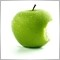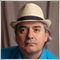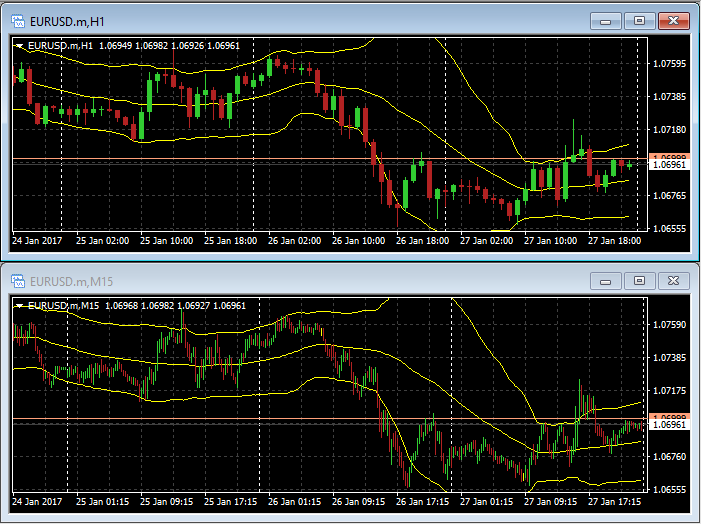# BBand settings?1562

When I use BBands period 20, dev 2.0 on M15, I change my timeframe to H1, That 20, 2.0 settings how changed on H1 timeframe?

Period 80? or else? And deviation 8.0? or else?Moderator
26132

Erdenebayar Lamjav:

When I use BBands period 20, dev 2.0 on M15, I change my timeframe to H1, That 20, 2.0 settings how changed on H1 timeframe?

Period 80? or else? And deviation 8.0? or else?

The settings stay the same1562

Keith Watford:
The settings stay the same

My question is wrong

BBands period 20, dev 2.0 on H1 is how about M15?Moderator
26132

Erdenebayar Lamjav:

My question is wrong

BBands period 20, dev 2.0 on H1 is how about M15?

The settings stay the same when you change time-frames.7155

I tell you a simple and intelligent way.

Put two indicator on chart with two setting that you need and in the Visualization tab of indicators setting window, select M15 for one and H1 for other.5110

Erdenebayar Lamjav: When I use BBands period 20, dev 2.0 on M15, I change my timeframe to H1, That 20, 2.0 settings how changed on H1 timeframe? Period 80? or else? And deviation 8.0? or else?
@All:
What the OP is asking about, is the use of the "equivalence ratio" of applying a moving average which will result in a similarity to a multi-time-frame indicator.
@Erdenebayar Lamjav
Yes Erdenebayar, you are partially correct! For a Bollinger Bands (which is based on a moving average), the period should be multiplied by the ratio between the two time-frames in order to compensate and obtain an equivalence.

So for a 20 Period Bollinger Band on H1, the equivalent on M15 is 20 x H1 / M15 = 20 * 60 / 15 = 20 * 4 = 80 (as you mentioned).

However, the Standard Deviation ratio should not be changed. It should remain at 2.0, as only the period needs to be adjusted.

Here is a screen shot to show the comparison, with the "Zoom" scale altered on the M15 so you can easily compare the two:1562

Fernando Carreiro:
@All:
What the OP is asking about, is the use of the "equivalence ratio" of applying a moving average which will result in a similarity to a multi-time-frame indicator.
@Erdenebayar Lamjav
Yes Erdenebayar, you are partially correct! For a Bollinger Bands (which is based on a moving average), the period should be multiplied by the ratio between the two time-frames in order to compensate and obtain an equivalence.

So for a 20 Period Bollinger Band on H1, the equivalent on M15 is 20 x H1 / M15 = 20 * 60 / 15 = 20 * 4 = 80 (as you mentioned).

However, the Standard Deviation ratio should not be changed. It should remain at 2.0, as only the period needs to be adjusted.

Here is a screen shot to show the comparison, with the "Zoom" scale altered on the M15 so you can easily compare the two:

Thank you Sir1464

Fernando Carreiro:
@All:
What the OP is asking about, is the use of the "equivalence ratio" of applying a moving average which will result in a similarity to a multi-time-frame indicator.
@Erdenebayar Lamjav
Yes Erdenebayar, you are partially correct! For a Bollinger Bands (which is based on a moving average), the period should be multiplied by the ratio between the two time-frames in order to compensate and obtain an equivalence.

So for a 20 Period Bollinger Band on H1, the equivalent on M15 is 20 x H1 / M15 = 20 * 60 / 15 = 20 * 4 = 80 (as you mentioned).

However, the Standard Deviation ratio should not be changed. It should remain at 2.0, as only the period needs to be adjusted.

Here is a screen shot to show the comparison, with the "Zoom" scale altered on the M15 so you can easily compare the two:

Well, it's not an equivalent (but can be said to be close enough), for example:

If you take the period on the 1 HR to be 1 (for simplicity) - and the 1 HR candle was closed supposedly on price 1.5
but the 1 HR candle is comprised of 4 15M candles that were closed in this order - 1.51, 1.53, 1.6, 1.5 (the average of those 4 candles is above 1.5 - so it's not equal the average of the 1HR)

on the long run it can be close enough yet, if you rely on the assumption that average of each 4 candles of the 15M will be approximately the same as the 1HR close price and if not then the flunctuation will be more or less the same - some candles will close above but then the next will close below and so on.